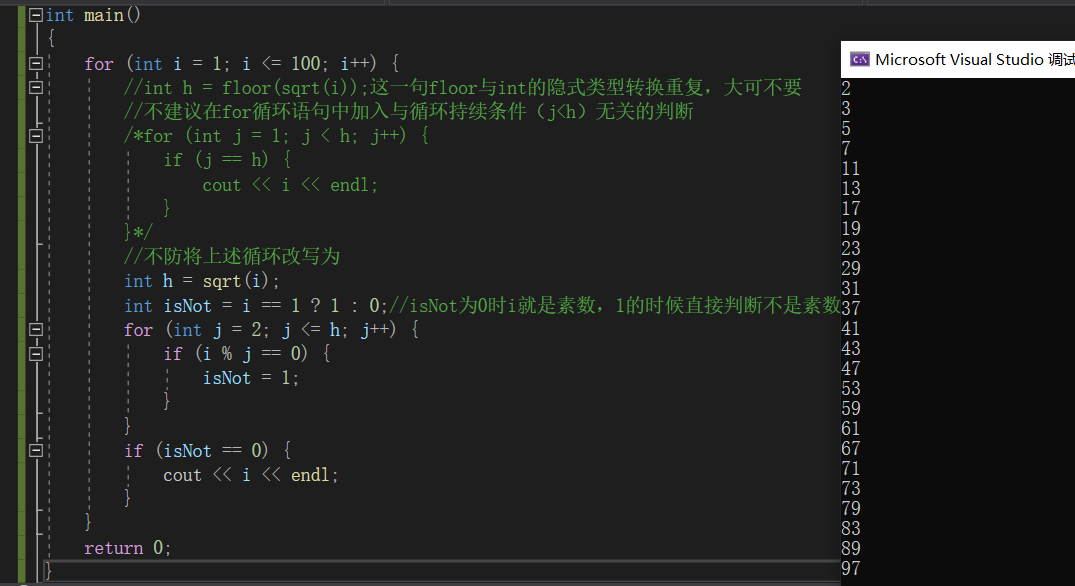``````#include <iostream>
#include <math.h>
#include <iomanip>
using namespace std;
int main()
{
for (int i = 1; i <= 100; i++) {
int h = floor(sqrt(i));
for (int j = 1; j <= h && i % j != 0; j++)
if (j == h + 1)
cout << i << endl;
}
return 0;
}

``````

3个回答

if (j = h)

``````#include <iostream>
#include <math.h>
#include <iomanip>
using namespace std;
int main()
{
for (int i = 2; i <= 100; i++) {
int h = floor(sqrt(i) + 0.1);
int j;
for (j = 2; j <= h && i % j != 0; j++);
if (j == h+1)
cout << i << endl;
}
return 0;
}

``````

2
3
5
7
11
13
17
19
23
29
31
37
41
43
47
53
59
61
67
71
73
79
83
89
978 个月之前 回复8 个月之前 回复azazinco 回复贵阳老马马善福专业维修游泳池堵漏防水工程: 为啥我把你的代码i和j的初始值改成1就输出不了
8 个月之前 回复8 个月之前 回复azazinco 我不是 cout << i << endl;了吗
8 个月之前 回复azazinco 回复贵阳老马马善福专业维修游泳池堵漏防水工程: 能分析一下我的代码为什么没有输出吗
8 个月之前 回复8 个月之前 回复azazinco 为啥我改成j==h还是没有输出
8 个月之前 回复8 个月之前 回复
``````#include <iostream>
#include <math.h>
using namespace std;
int main()
{
for (int i = 1; i <= 100; i++) {
//int h = floor(sqrt(i));这一句floor与int的隐式类型转换重复，大可不要
//不建议在for循环语句中加入与循环持续条件（j<h）无关的判断
/*for (int j = 1; j < h; j++) {
if (j == h) {
cout << i << endl;
}
}*/
//不防将上述循环改写为
int h = sqrt(i);
int isNot = i == 1 ? 1 : 0;//isNot为0时i就是素数，1的时候直接判断不是素数
for (int j = 2; j <= h; j++) {
if (i % j == 0) {
isNot = 1;
}
}
if (isNot == 0) {
cout << i << endl;
}
}
return 0;
}

``````loop{break now} 回复azazinco: 你还是没搞明白~~如果j是1，那么if(i%1==0)永远满足条件，isNote永远为1，永远不是素数，永远没的输出，素数的概念不要搞错，是出了1和其本身不再可以被其他的数整除
8 个月之前 回复azazinco 回复與蘤舊縐: 吧int j = 2改成int j = 1
8 个月之前 回复loop{break now} 回复azazinco: 我不本来就是int j=2么
8 个月之前 回复azazinco 回复與蘤舊縐:把你的代码改成int j = 2就输出不了
8 个月之前 回复loop{break now} 回复azazinco: 不存在的~~，你是不是漏了什么，我贴个图
8 个月之前 回复azazinco 我运行后是空的
8 个月之前 回复azazinco 好吧有问题，但是为什么cout << i << endl没有输出
8 个月之前 回复C语言计算1000以内的质数的和，并且输出出来，代码

C 语言计算1000以内的质数的和，并且输出出来，代码怎么来写

for (int i = 101; i <=200; i++) { for (int j = 2; j < j/2; j++) { if (i % j == 0) { System.out.println(i + "是素数"); } } } 这个怎么没有输出呢，我的代码应该没问题吧

#include<iostream> #include<math.h> #include<string> using namespace std; class Prime { private: int a; int n1,n2; int num; public: Prime(int n1,int n2,int num); void primef(); void show(); }; Prime::Prime(int m1,int m2,int n):n1(m1),n2(m2),num(n){} void Prime::primef() { int m,i,k,leap=1,h=0; for(m=100;m<=200;m++) { k=sqrt(m); for(i=2;i<=k;i++) { if(m%i==0) { leap=0; } } if(leap==1) { a[h]=m; h=h+1; num=1+num; } } cout<<num; } void Prime::show() { int j; for(j=0;j<=(num-1);j++) { cout<<"a[j]="<<a[j]<<" "; if(j%5==0) cout<<endl; } cout<<"num="<<num<<endl; } int main() { Prime p(100,200,0); p.primef(); p.show(); return 0; }

c语言求1~n之间所有素数的和

Problem Description Give you a lot of positive integers, just to find out how many prime numbers there are. Input There are a lot of cases. In each case, there is an integer N representing the number of integers to find. Each integer won’t exceed 32-bit signed integer, and each of them won’t be less than 2. Output For each case, print the number of prime numbers you have found out. Sample Input 3 2 3 4 Sample Output 2

C++用筛法求100内素数

#include "stdio.h" void main() { int i,j,flag; for(j=100;j<=200;j++) { for(i=2;i<j;i++) flag=1; if(j%i==0) { flag=0; i=j; } if(flag==1) printf("%d为素数\n",j); else printf("%d不为素数\n",j); } }

Java语言悬赏问题，怎么计算1000以内所有的回文数

Java语言悬赏问题，怎么计算1000以内所有的回文数 Java语言悬赏问题，怎么计算1000以内所有的回文数

Java基础知识面试题（2020最新版）

Intellij IDEA 实用插件安利

1. 前言从2020 年 JVM 生态报告解读 可以看出Intellij IDEA 目前已经稳坐 Java IDE 头把交椅。而且统计得出付费用户已经超过了八成（国外统计）。IDEA 的...

MySQL数据库面试题（2020最新版）

2020阿里全球数学大赛：3万名高手、4道题、2天2夜未交卷

HashMap底层实现原理，红黑树，B+树，B树的结构原理 Spring的AOP和IOC是什么？它们常见的使用场景有哪些？Spring事务，事务的属性，传播行为，数据库隔离级别 Spring和SpringMVC，MyBatis以及SpringBoot的注解分别有哪些？SpringMVC的工作原理，SpringBoot框架的优点，MyBatis框架的优点 SpringCould组件有哪些，他们...

《Oracle Java SE编程自学与面试指南》最佳学习路线图2020年最新版（进大厂必备）